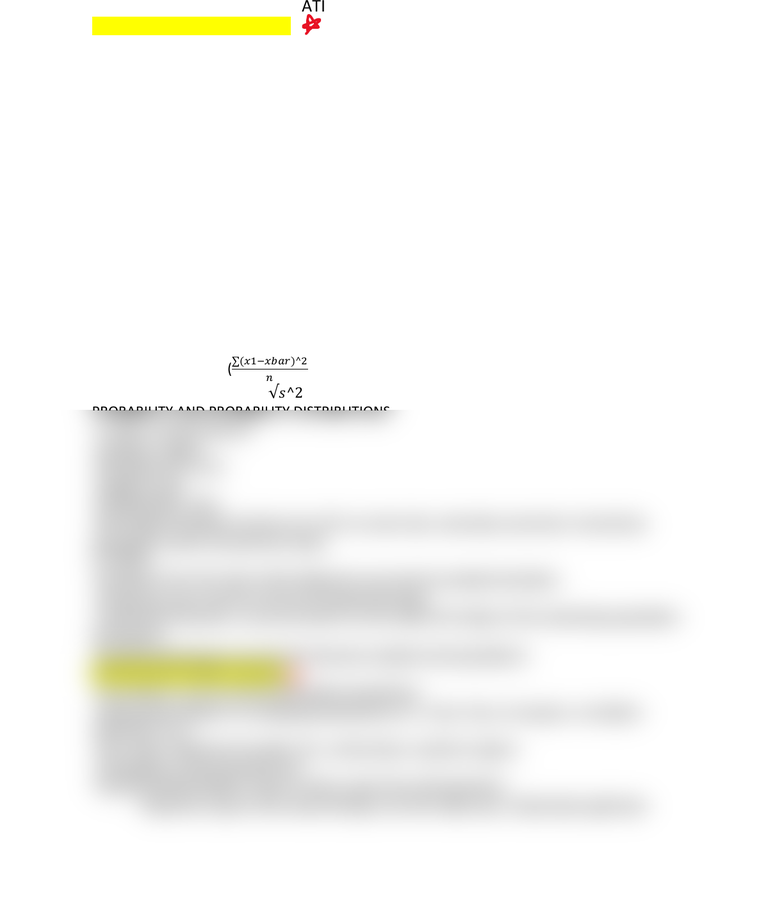Class Notes (1,100,000)
US (470,000)
UMD (8,000)
CCJS (100)
Lecture 7

# CCJS 300 Lecture Notes - Lecture 7: Central Limit Theorem, Null Hypothesis, Chi-Squared Distribution

Department
Criminology and Criminal Justice
Course Code
CCJS 300
Professor
Alan Lehman
Lecture
7

This preview shows half of the first page. to view the full 1 pages of the document.STATISTICS & DATA ANALYSIS
-Each step of hypothesis testing
-CCJS is a science, statistics is a tool we use to answer our questions
DESCRIPTIVE AND INFERENTIAL RESEARCH
-Explanatory research
-Language & vocabulary
-Theories, hypotheses, validity, causality
-Sampling
-Independent & dependent variables
-Levels of measurement (nominal, ordinal-order the responses, interval-equal intervals
between, ratio- true zero point)
-Measures of central tendency:
-Mode: most frequently seen actual number
-Median: middle value in a set of data, the 50th percentile
-Mean: the average of a set of data (subject to influence by outliers)
-VR: variation ratio, 1-(fmodal category/n)
-Range: Highest value-lowest value
-IQR: interquartile range, middle 50% of a distribution
-Variance: s2= (
)
-Standard deviation:
PROBABILITY AND PROBABILITY DISTRIBUTIONS
-1 trial vs “in the long run
-Gambler’s fallacy
-Bounding rule 0<p<1
-Multiplication rules
-Bell shaped standard normal curve, 50% on each side, unimodal, symmetric, theoretical,
asymptotic (never touches the x-axis)
Z SCORES
-Deviation from the mean while taking into account the standard deviation
-Going from raw scores to scores in its particular data
-Central limit theorem: we do not have to worry about the shape of the underlying population
distribution
-Sampling distributions are the link between samples and populations
STEPS IN HYPOTHESIS TESTING
-Associations, set up null and alternative hypotheses
-Appropriate statistic & its sampling distribution (i.e. t test, f test, chi square, correlation
regression, etc.)
-Set p value, alpha level (usually .05), critical value, rejection regions
-Calculations, performing the test
-Decision/Interpretation, reject or fail to reject the null hypothesis
-Rejection is good, this means finding is not the status quo, statistically significant